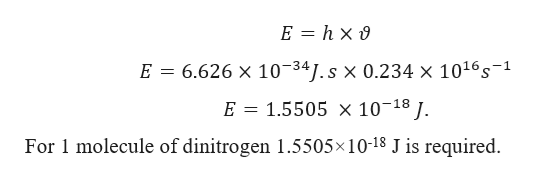# A light photon with a frequency of at least 0.234 × 1016 Hz is required to split apart dinitrogen according to the equation:N2(g) → 2N(g)Calculate the energy (kJ) required to break apart a mole of dinitrogen. Express answer in scientific notation.

Question
1 views
 A light photon with a frequency of at least 0.234 × 1016 Hz is required to split apart dinitrogen according to the equation: N2(g) → 2N(g) Calculate the energy (kJ) required to break apart a mole of dinitrogen. Express answer in scientific notation.
check_circle

Step 1

Given:

Frequency = 0.234 × 1016 Hz = 0.234 × 1016 s&minu...help_outlineImage TranscriptioncloseE hx 6.626 x 10-341.s x 0.2 34 x 1016s-1 E 1.5505 x 10-18 I. E For 1 molecule of dinitrogen 1.5505x 10-18 J is required fullscreen

### Want to see the full answer?

See Solution

#### Want to see this answer and more?

Solutions are written by subject experts who are available 24/7. Questions are typically answered within 1 hour.*

See Solution
*Response times may vary by subject and question.
Tagged in

### Atomic structure